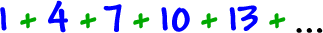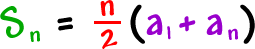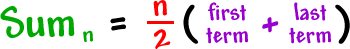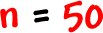A similar formula works for when the terms skip some numbers, likeHere's the formula:

 To find the sum of the first n terms:Here's how I remember it:* This is for arithmetic sequences ONLY!

Let's try it!

Let's find the sum of the first 50 terms of the arithmetic sequence:We have:We need: